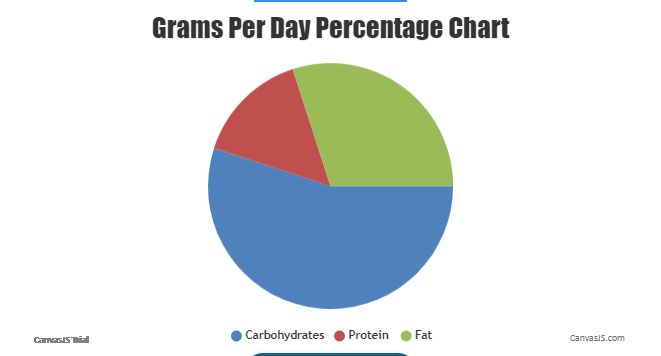Uh Oh! It seems you’re using an Ad blocker!

We always struggled to serve you with the best online calculations, thus, there's a humble request to either disable the AD blocker or go with premium plans to use the AD-Free version for calculators.

Or# Harris Benedict Equation Calculator

Age Years:

Gender:

Height:

Height (cm):

Weight:

PAL (optional):

Table of Content

 1 What is priceeight Class? 2 priceeight Class Chart: 3 How to Calculate priceeight Density (Step by Step): 4 Factors that Determine priceeight Classification: 5 What is the purpose of priceeight Class? 6 Are mentioned priceeight Classes verified by the officials? 7 Are priceeight Classes of UPS and FedEx same?

Get the Widget!

Add this calculator to your site and lets users to perform easy calculations.

Feedback

How easy was it to use our calculator? Did you face any problem, tell us!

Our Harris benedict calculator calculates the total number of calories needed in your diet using the Harris benedict equation. With the help of this calculator, you can measure your BMR, total energy expenditure, and the macronutrients you need to consume daily.

## How does This Harris Benedict Calculator Work?

Follow the below-mentioned steps to use this Harris-benedict equation calculator:

Input:

• Enter your height and weight.
• Hit the “Calculate” button.

Output:

• The BMR level
• TDEE value
• Macronutrients

## What is the Harris-Benedict Equation?

The first formula to measure BMR was invented by Harris-Benedict. The Harris-Benedict formula calculator is based on the variables like height, weight, age, gender, and activity level. You can calculate the Harris benedict formula manually or by using a Harris benedict calculator.

Harris benedict formula calculator uses different equations for men and women:

Sex Units Calculation
Men Metric BMR = 66.5 + ( 13.76 × weight in kg ) + ( 5.003 × height in cm ) – ( 6.755 × age in years )
Imperial BMR = 66 + ( 6.2 × weight in pounds ) + ( 12.7 × height in inches ) – ( 6.76 × age in years )
Women Metric BMR = 655 + ( 9.563 × weight in kg ) + ( 1.850 × height in cm ) – ( 4.676 × age in years )
Imperial BMR = 655 + ( 4.35 × weight in pounds ) + ( 4.7 × height in inches ) – ( 4.7 × age in years )

## How to Calculate the TDEE Using Harris-benedict?

You can easily calculate the total energy expenditure by multiplying your BMR with various activity factors or simply use a Harris benedict tdee calculator.

• For sedentary, calculate the calories by multiplying the BMR by 1.2 (Calories = BMR * 1.2).
• For lightly active sports, multiply your BMR by 1.375 (Calories = BMR * 1.375).
• If you’re moderately active, calculate your calories by multiplying your BMR by 1.55 (BMR * 1.55).
• If you’re very active (Hard exercise), you can calculate your calories by multiplying the BMR by 1.725 (calories = BMR * 1.725).
• Lastly, if you are extra active then calculate your calories by the following formula: calories = BMR * 1.9

Here, you can see the appropriate chart of calculating the TDEE.

Calorie-Calculation Sedentary Little or No Exercise BMR x 1.2 Sports 1-3 Days/Week BMR x 1.375 Sports 3-5 Days/Week BMR x 1.55 Sports 6-7 Days a Week BMR x 1.725 Physical Job or 2xTraining BMR x 1.9

Note: If you don’t want to do it manually, you can simply use our Harris benedict equation calculator.

## Practical Example:

Calculate the BMR, TDEE, and Macros requirement of a person. The age of the person is 25 years his height is 5 feet 9 inches, his weight is 158 lbs and he is maintaining a moderate activity level.

#### Given:

Age = 25 years

Gender = Male

Height =  5 feet 9 inches

Weight = 158 lbs

Activity level = Moderate

#### Solution:

Enter values in the harris-benedict equation for men:

BMR = 66.5 + (13.75 × weight [kg]) + (5.003 × height [cm]) – (6.775 × age [years])

BMR =1760 kcal/day

Total Energy Expenditure =2728 kcal/day

Carbohydrates =375 Grams per day

Protein = 102 Grams per day

Fats = 205 Grams per dayWhen you are using the Harris benedict equation calculator, then you know the accurate calorie deficit, according to your activity level and body weight. The Harris benedict calculator precise values of BMR and TDEE relative to the activity level, height, weight, age, and gender.

## FAQs:

### How do I Calculate my BMR?

You can use the Harris benedict equation or Harris-benedict calculator to calculate your BMR.

• For Men: BMR = 66.5 + (13.75 × weight in kg) + (5.003 × height in cm) – (6.75 × age)
• For Women: BMR = 655.1 + (9.563 × weight in kg) + (1.850 × height in cm) – (4.676 × age)

### What is a Good BMR?

Men and women have different BMRs for men, the good BMR is 1409 kcal and for women, the good BMR is 1696 kcal. And the BMR can be measured using a Harris benedict bmr calculator.

### How do I Increase My BMR?

It’s really simple, follow the below-mentioned steps:

• Consume more protein in every meal.
• Have some more spicy foods.
• Do more workouts.
• Increase your daily sports activity.

### References:

From the source Wikipedia: Harris-Benedict equation,The Harris-Benedict BMR

From the source ncbi.nlm.nih.gov: Harris Benedict formula,Predict energy requirements